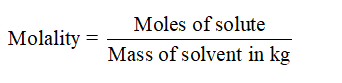# Find the molality of a solution in which the benzene (C6H6) to toluene (C7H8) molar ratio is exactly 4:1.

Question
14 views
Find the molality of a solution in which the benzene (C6H6) to toluene (C7H8) molar ratio is exactly 4:1.

check_circle

Step 1

The molality of a solution is to be determined in which the benzene (C6H6) to toluene (C7H8) molar ratio is exactly 4:1.

Step 2

The molality of a solution is defined as the moles of solute per kg of solvent.Step 3

Now, the molar ratio of benzene to toluene is 4:1.

Thus, assume the moles of benzene to be 4 moles and moles of toluene to be 1 mole.

A...

### Want to see the full answer?

See Solution

#### Want to see this answer and more?

Solutions are written by subject experts who are available 24/7. Questions are typically answered within 1 hour.*

See Solution
*Response times may vary by subject and question.
Tagged in

### Chemistry